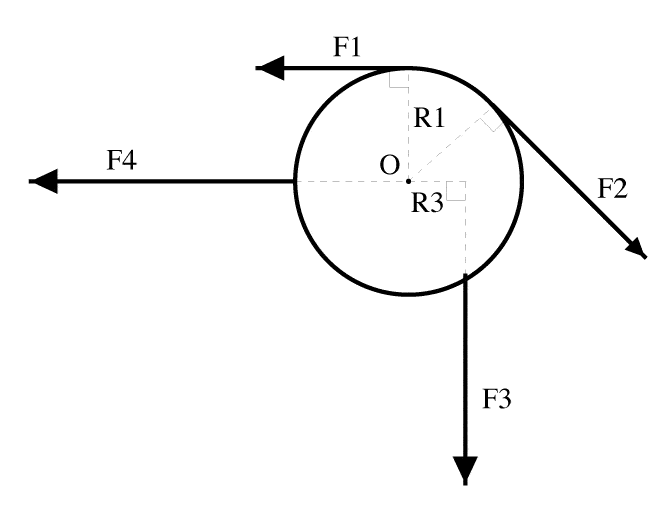# Rotational Motion of a Cylinder

## Homework Statement

A uniform 3.8-kg cylinder can rotate about an axis through its center at O. The forces applied are: F1 = 4.6 N, F2 = 6.2 N, F3 = 8.0 N, and F4 = 3.1 N. Also, R1 = 10.7 cm and R3 = 5.8 cm. Find the magnitude and direction (+: counterclockwise; -: clockwise) of the angular acceleration of the cylinder.

F1 = 4.6N
F2 = 6.2N
F3 = 8.0N
F4 = 3.1N

R1 = .107M
R2 = .107M
R3 = .058M
R4 = .107M

I = .2033
M= 3.8kgT = F * R
ang accel = T/I
I= 1/2MR^2

## The Attempt at a Solution

*0 = omega since i don't have key
T=Frsin0
F1= 4.6*.107sin90 = .4922
F2= 6.2*.107sin90 = -.6634
F3= 8.0*.058sin90 = -.464
F4= 0
Fnet = -.6352

## Homework Statement

A uniform 3.8-kg cylinder can rotate about an axis through its center at O. The forces applied are: F1 = 4.6 N, F2 = 6.2 N, F3 = 8.0 N, and F4 = 3.1 N. Also, R1 = 10.7 cm and R3 = 5.8 cm. Find the magnitude and direction (+: counterclockwise; -: clockwise) of the angular acceleration of the cylinder.

F1 = 4.6N
F2 = 6.2N
F3 = 8.0N
F4 = 3.1N

R1 = .107M
R2 = .107M
R3 = .058M
R4 = .107M

I = .2033
M= 3.8kg

T = F * R
ang accel = T/I
I= 1/2MR^2

## The Attempt at a Solution

*0 = omega since i don't have key
T=Frsin0
F1= 4.6*.107sin90 = .4922
F2= 6.2*.107sin90 = -.6634
F3= 8.0*.058sin90 = -.464
F4= 0
Fnet = -.6352